• Introduction
• What is in this manual
• What is Caspoc
• User interface
• Introduction
• Starting
• Simulation
• Editing
• Viewing and printing
• Getting Started
• Basic editing
• Simulation in the time domain
• Basic User Interface Topics
• Editing
• Simulation
• Viewing
• Library
• Reports
• Project management
• Circuit and Block Diagram Components
• Introduction
• Cscript and user defined functions
• Component parameters
• Modeling Topics
• Introduction
• Power Electronics
• Semiconductors
• Electrical Machines
• Electrical drives
• Power Systems
• Mechanical Systems
• Thermal Systems
• Magnetic Circuits
• Green Energy
• Coupling to FEM
• Experimenter
• Analog hardware description language
• Embedded C code Export
• Coupling to Spice
• Small Signal Analysis
• Matlab coupling
• Tips and tricks
• Appendices

## Create your first rectifier simulation

In this tutorial we will create a simple single phase rectifier. It will be a single phase rectifier connected to the mains. Create the circuit as shown below. Select the voltage source from Components/Circuit/Sources and the diode from Components/Circuit/Semiconductor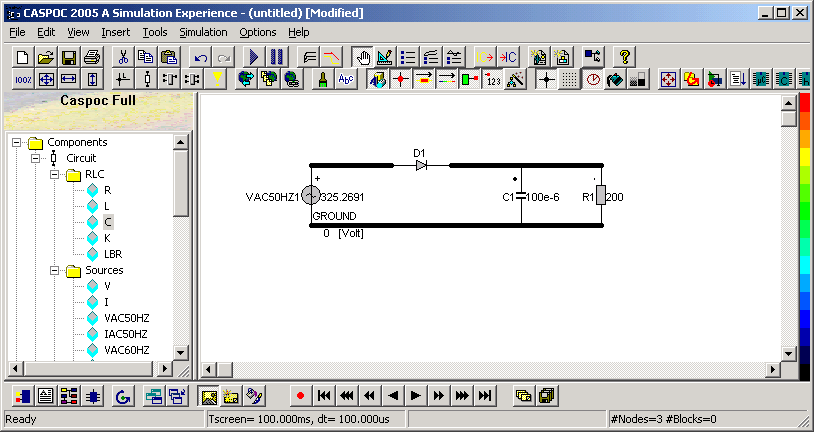We want to display both the output voltage and the diode current in one scope. Select a scope from the buttunbar at the bottom of your window.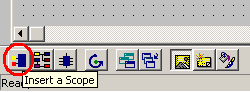Enlarge the scope to create more inputs. We want to display the output voltge and diode current, so two inputs are required. Click and drag the bottom-right corner of the scope with the left mouse button clicked. During the resizing of the scope, the number of inputs will be shown.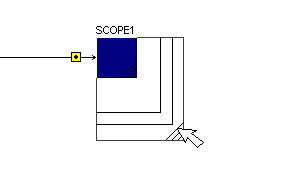If the input from a scope is over a node, its input connection is displayed as a square. The voltage on that node will be displyed in the scope.
To draw a current in the scope, drag the input of the scope over the component that is used for current measurement. Click the input of the scope with the left mouse button and drag it over the component. Release the left mouse button over the circuit component and a dotted line is drawn from the component to the input of the scope, indicating that the current through that component is displayed in the scope.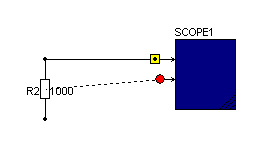Draw a wire from the output of the rectifier to the second input of the scope and drag the first input of the scope over the diode.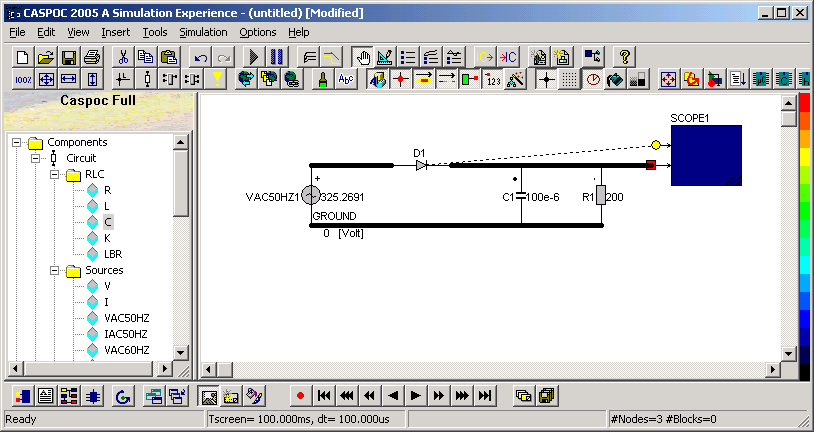Next we have to define the parameters for the simulation time. We have to set the total simulation time, the simulation step size and the numerical method. Open the simulation parameters dialog box by selecting Simulation/Simulation Parameters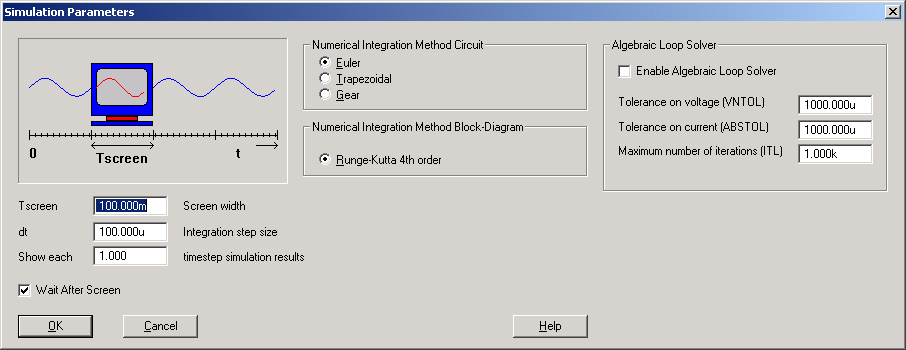The parameters that we have to set here are:
• TscreenThe total simulation time that will be simulated.
• dtThe step size. Each dt simulation results are displayed in the scope. Select dt small enough, for example, 100 to 1000 steps per period.
• Numerical integration method select the Euler numerical integration method for this example.
First we set the parameter Tscreen. Since our voltage source has a frquency of 50Hz, we select 5 periods to be simulated. The parameter Tscreen is thus set to 5*20ms = 100ms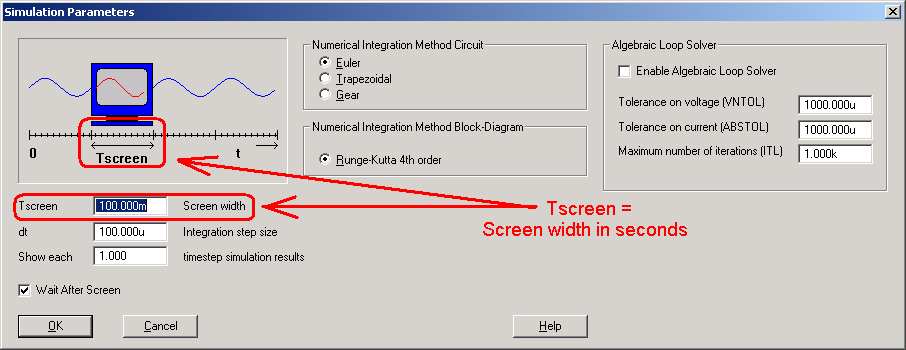Second we set the step size parameter dt for this simulation. The period of the voltage source is 20ms. The diode will conduct for only a small portion of that period. Therefore we select a small step size of 100us for this simulation.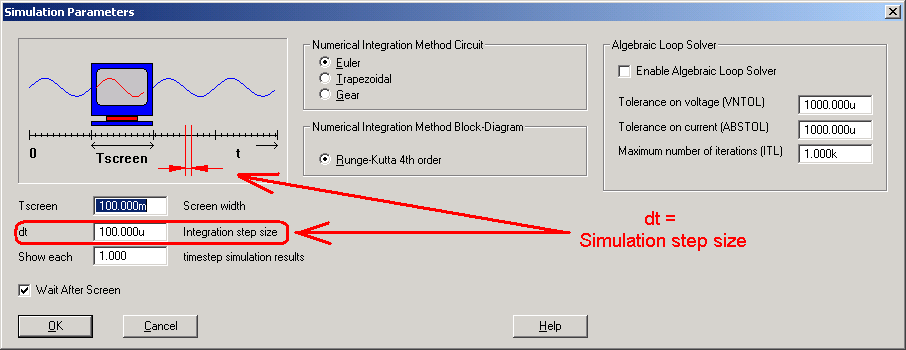The last parameter is the numerical method. Select Euler, which is a stabil method.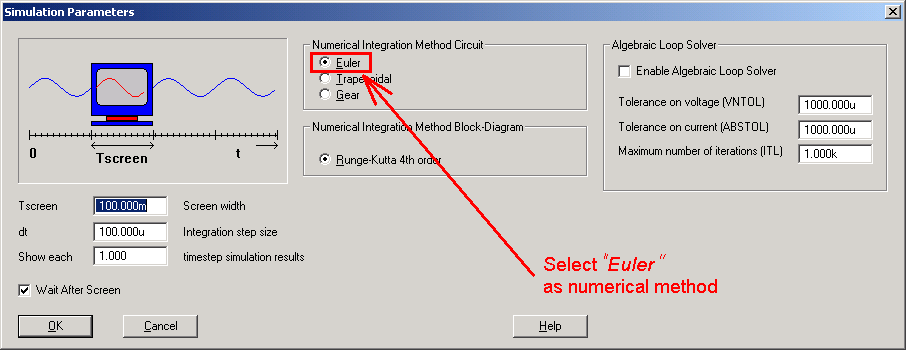Start the simulation by pressing the Play button or pressing the [Enter] key. The simulation is now started and the output voltage and the current through the diode are shown in the scope. Open the scope by clicking it with the right mouse button.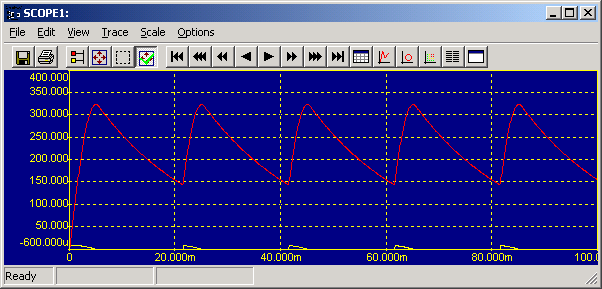The output voltage shows a large ripple. To reduce the size of the ripple we can increase the value of the output capacitor. Open the properties dialog box for the capacitor by clicking it with the right mouse button and enter the value of 1mF at the field for the value of the capacitor.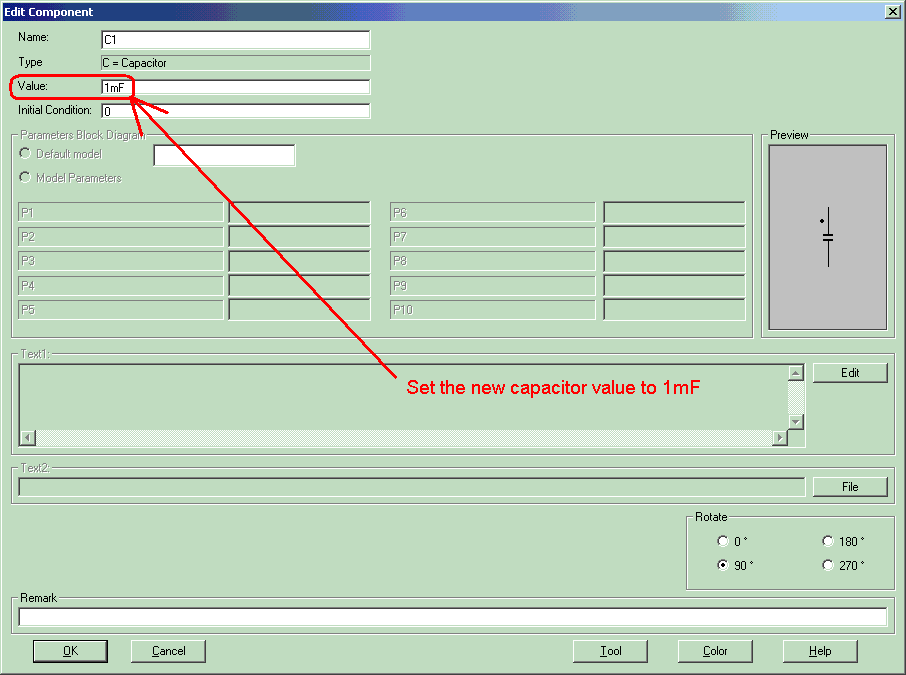Restart the simulation by pressing the Play button or continue the simulation by pressing the [Enter] key. The simulation is now started and the output voltage and the current through the diode are shown in the scope.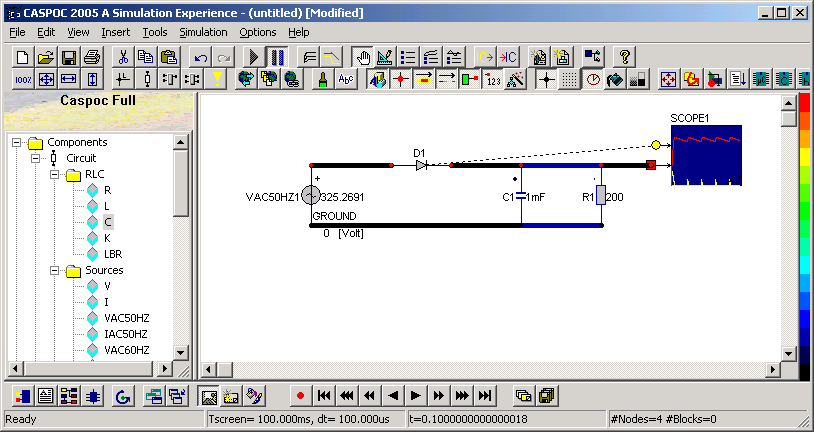Open the scope by clicking it with the right mouse button.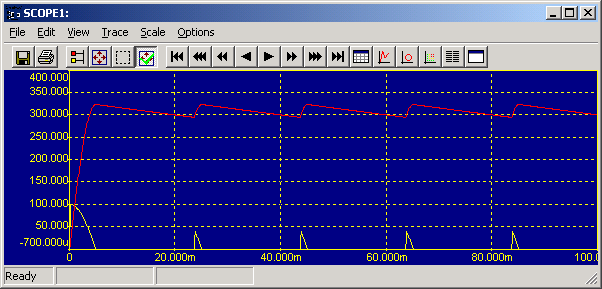We also want to display the input voltage. Therefore we increase the size of the scope to increase the number of inputs to three.We move the scope such that the third input is over the node for the output voltage. From the input node we draw a wire to the first input of the scope.
Restart the simulation by pressing the Play button or continue the simulation by pressing the [Enter] key. The simulation is now started and the output voltage and the current through the diode are shown in the scope.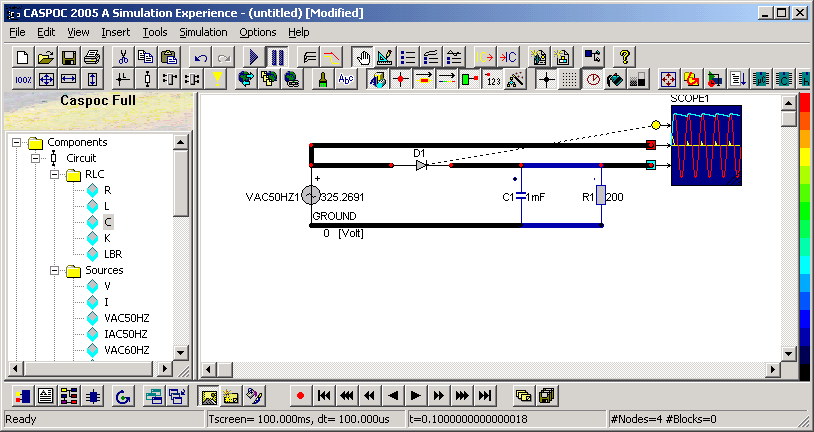Open the scope by clicking it with the right mouse button.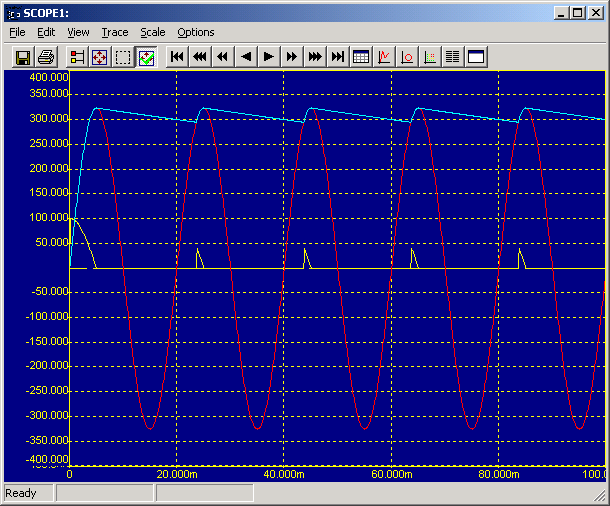The scope shows the input voltage(red trace), the output voltage(blue trace) and the current through the diode(yellow trace). Note that the current flows into a component following the dot convention. The current through a voltage source is positive if it flows from the positive node through the voltage source to the negative node. Therefore the measured current through our voltage source is indicated to be negative.